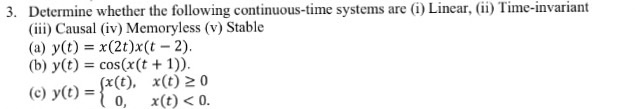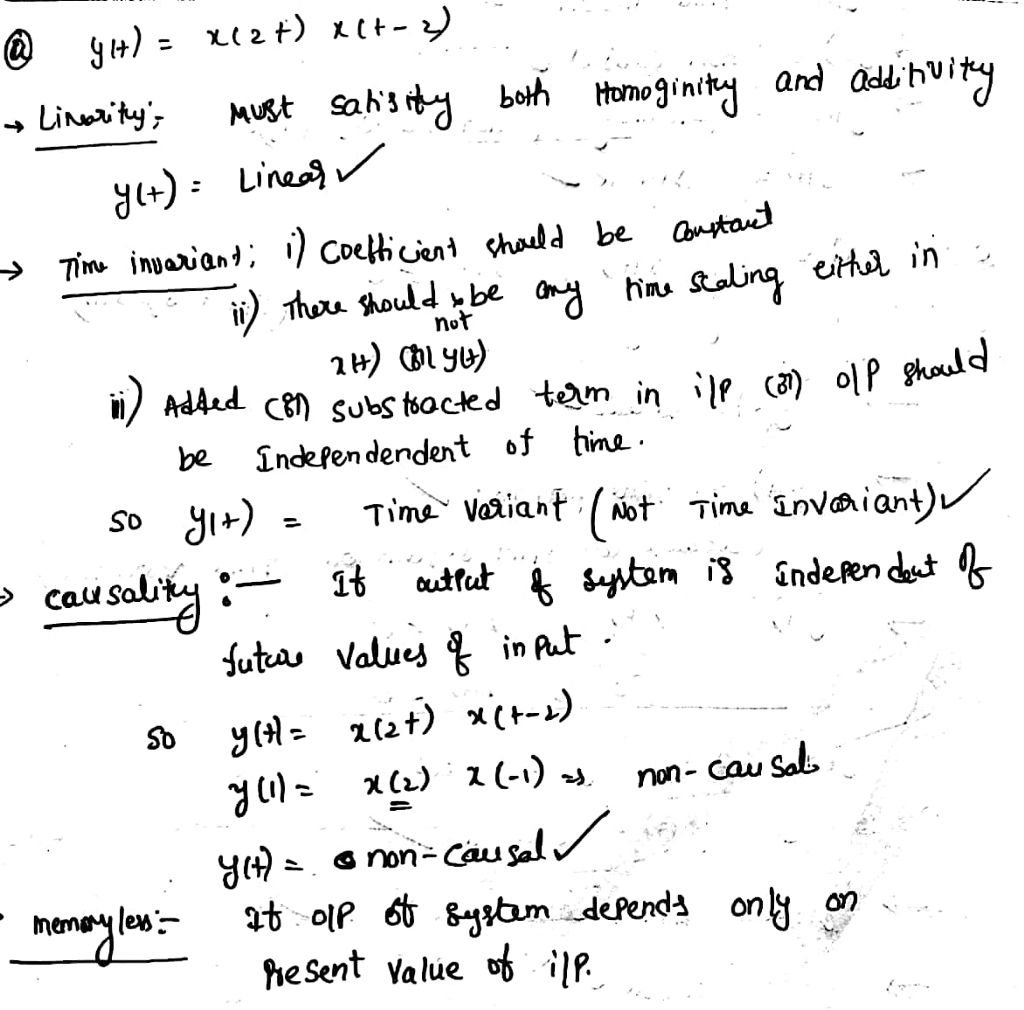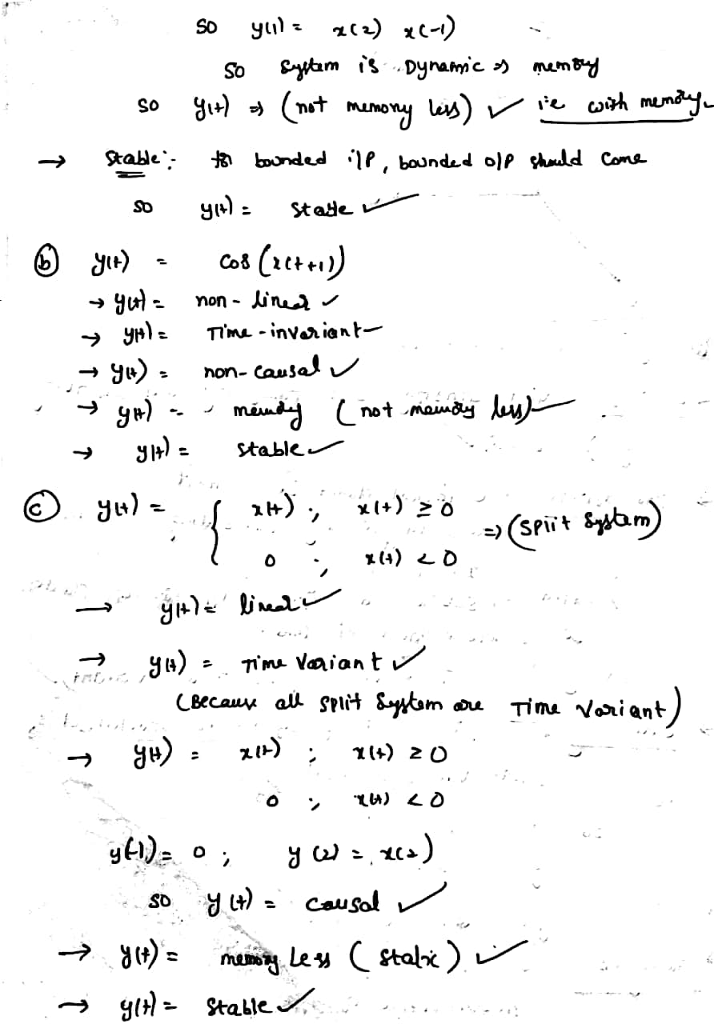# Homework Solution: Determine whether the following continuous-time systems are (i) Linear, (i) Time-invariant (ii) Causal (iv)…Determine whether the following continuous-time systems are (i) Linear, (i) Time-invariant (ii) Causal (iv) Memoryless (v) Stable (a) y(t) = x(2t)x(t - 2). (b) y(t) = cos(x(t + 1)). (c) y(t) = {x(t), x(t) greaterthanorequalto 0 0, x(t)